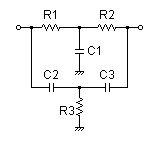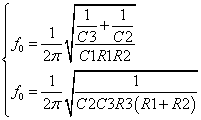# Twin-T Notch Filter Design Tool

This page is a web application that design a Twin-T notch filter. Use this utility to simulate the Transfer Function for filters at a given center rejection frequency or values of R and C. The response of the filter is displayed on graphs, showing Bode diagram, Nyquist diagram, Impulse response and Step response.

## Calculate the transfer function for Twin-T notch filter with R and C values

 Vin(s)→→Vout(s)
Transfer function
Transfer function of Twin-T notch filter
 R1=Ω C1=F R2=Ω C2=F R3=Ω C3=F
p:pico, n:nano, u:micro, k:kilo, M:mega

### Frequency analysis

Bode diagram
Phase  Group delay
Nyquist diagram
Pole, zero
Phase margin
Oscillation analysis

Upper and lower frequency limits:
f1= - f2=[Hz] (frequency limits are optional)

### Transient analysis

Step response
Impulse response
Overshoot
Final value of the step response

Simulation time:
0 - [sec] (optional)

## Calculate the R and C values for the Twin-T notch filter at a given frequency

 Vin(s)→→Vout(s)
Center rejection frequencyf0= Hz
 C1 = F C2 = F C3 = F
C1, C2, C3 is optional. But when setting these capacitances, C1, C2 and C3 of all are needed to give.

Select Capacitor Sequence:
Select Resistor Sequence:

### Frequency analysis

Bode diagram
Phase  Group delay
Nyquist diagram
Pole, zero
Phase margin
Oscillation analysis

Upper and lower frequency limits:
f1= - f2=[Hz] (frequency limits are optional)

### Transient analysis

Step response
Impulse response
Overshoot
Final value of the step response

Simulation time:
0 - [sec] (optional)